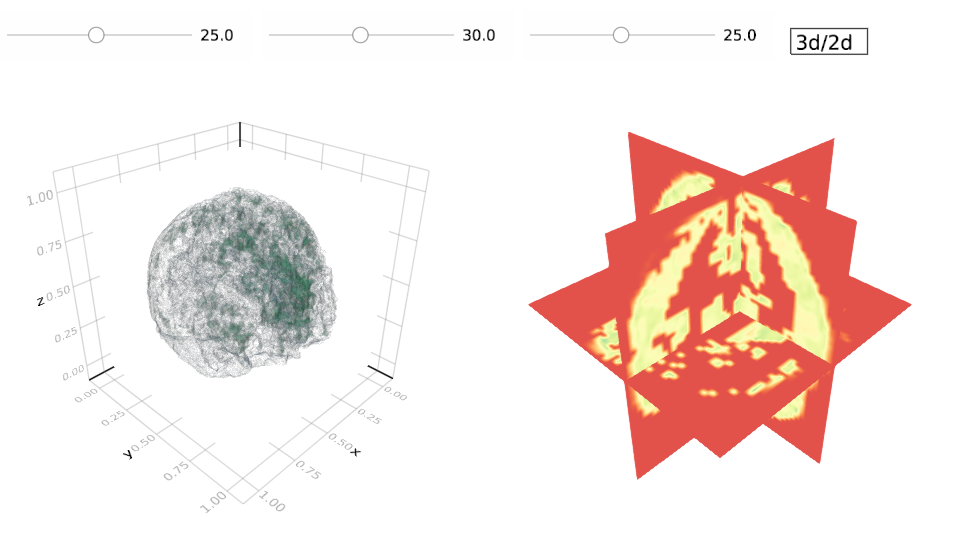# FMRI visualization with Julia

# you may add more subject if you want to investigate
for i in 1:2
download("http://www.cs.cmu.edu/afs/cs/project/theo-73/www/plosone/files/subject_$i.mat", "subject_$i.mat")
end

2-Installing MAT.jl for Julia1.0

using Pkg
pkg"add https://github.com/halleysfifthinc/MAT.jl#v0.7-update"

3-Include required packages

using Makie, GeometryTypes
using AbstractPlotting: slider!, playbutton, vbox, hbox!
using Observables
using MAT

subject_id   = 1
data = subject["data"];

Time Steps

T = size(data,1)

Voxel Count

V = size(data,2)

colToCoord is array of voxel locations. Given index of voxel you get the location

colToCoord = vec(mapslices(x->CartesianIndex(Tuple(Int.(x))),subject["meta"]["colToCoord"];dims=2));

Sizes of the cube that includes all voxels

lx,ly,lz=maximum(Int.(subject["meta"]["colToCoord"]);dims=1);

FMRI Data Array: each index corresponds to a time and the value is the FMRI data taken at that time.

fmri_time_series = map(1:T) do i
voxels = zeros(lx,ly,lz)
voxels[colToCoord] = data[i,:];
voxels
end;
typeof(fmri_time_series)

Normalize Data

fm = fmri_time_series
mini, maxi = mapreduce(extrema, (a, b)-> (min(a, b), max(a, b)), fm)
fm_n = map(fm) do vol
Float32.((vol .- mini) ./ (maxi - mini))
end
ex_volume = first(fm_n);

Makie Visualization

9.4s
Language:Julia
style = Theme(raw = true, camera = campixel!)
#TIME Slider
tslider = slider(style, 1:length(fm_n))
t = tslider[end];
r = range(-1, stop = 1, length = size(ex_volume, 1))
r2 = range(0, stop = 1, length = size(ex_volume, 1))
scene3d = contour(r2, r2, r2, ex_volume, alpha = 0.1, levels = 4)
c = scene3d[end];

#c is unintuitive, is there a function to get the observable value of contour object  ?
volume = c
planes = (:xy, :xz, :yz)
hscene = Scene(camera = cam3d!, show_axis = false)
sliders = ntuple(3) do i
sscene = slider(style, 1:size(volume[], i), start = size(volume[], i) ÷ 2)
s = sscene[end]
idx = s[:value]; plane = planes[i]
indices = ntuple(3) do j
planes[j] == plane ? 1 : (:)
end
hmap = heatmap!(
hscene, r, r, volume[][indices...], colormap = :Spectral, fillrange = true,
interpolate = true, colorrange = (0, 1)
)[end]
lift(idx, volume) do _idx, vol
idx = (i in (1, 2)) ? (size(vol, i) - _idx) + 1 : _idx
transform!(hmap, (plane, r[_idx]))
indices = ntuple(3) do j
planes[j] == plane ? idx : (:)
end
if checkbounds(Bool, vol, indices...)
hmap[] = view(vol, indices...)
end
end
sscene
end

lift(t[:value]) do idx
if checkbounds(Bool, fm_n, idx)
c[] = fm_n[idx]
end
end

b2 = button(style, "3d/2d"; dimensions = (60, 40))
on(b2[end][:clicks]) do clicks
if iseven(clicks)
cam3d!(hscene)
else
cam2d!(hscene)
end
center!(hscene)
end
hbox(
vbox(scene3d, hscene),
vbox(sliders..., b2)
)